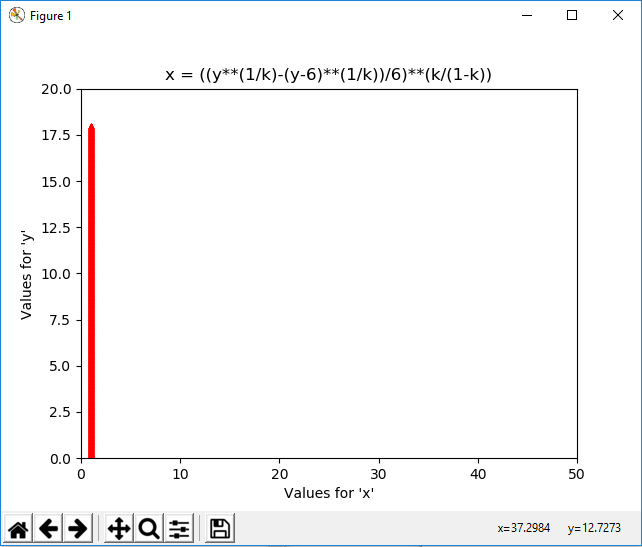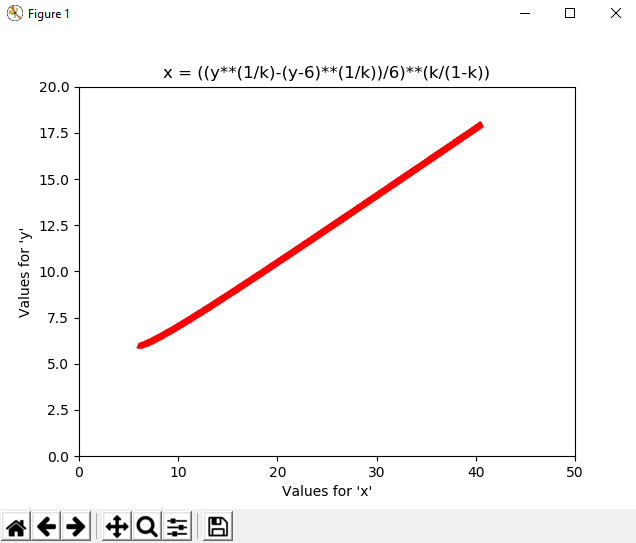Bottom Page

• 0 Vote(s) - 0 Average
• 1
• 2
• 3
• 4
• 5
 Solving Equations with PythonnewbieAuggie2019SplitterPosts: 195 Threads: 19 Joined: Aug 2019 Reputation: 10 Likes received: 21 #9 Sep-13-2019, 02:29 AM (This post was last modified: Sep-13-2019, 06:13 AM by newbieAuggie2019. Edited 2 times in total.) I just modified the program to format a bit the output on matplot: ```import numpy as np import matplotlib.pyplot as plt x = np.arange(4,12,.01) bigx = len(x) print("\nThe matrix 'x' has", bigx, "elements.") y = np.arange(0,18,.01) ynumbers = [y] bigy = len(y) print("The matrix 'y' has", bigy, "elements.") mother_of_matrices = bigx*bigy print("Therefore, the interaction of matrices 'x' and 'y' produces a huge matrix of:", mother_of_matrices, "elements.") k = 10/(6+x) print('\n\nThese are the values for "x":\n\n', x) print('\n\nThese are the values for "y":\n\n', y) print('\n\nThese are the values for "k":\n\n', k) for y in ynumbers: validynumbers = list(filter(lambda k: (1-k) != 0 and k != 0, k)) for k in k: x = ((y**(1/k)-(y-6)**(1/k))/6)**(k/(1-k)) plt.scatter(x, y, s=10, c='red', marker='^') # s: size of point, default = 20 # c: color, sequence, or sequence of color, default = ‘b’ (blue) # marker: point symbol, default = 'o’ plt.xlim(0,50) plt.ylim(0,20) plt.title('x = ((y**(1/k)-(y-6)**(1/k))/6)**(k/(1-k))') plt.xlabel("Values for 'x'") plt.ylabel("Values for 'y'") plt.show() ```This version of the program, as I said in a previous post, produces a first ouput in matplot that I attach here:where it seems that the values for 'y' are correct, going from '0' to '18' as indicated by line 7: `y = np.arange(0,18,.01)`but the values for 'x' have been changed all to '1'. The formatting lines for the matplot output are these lines (25-35): ```plt.scatter(x, y, s=10, c='red', marker='^') # s: size of point, default = 20 # c: color, sequence, or sequence of color, default = ‘b’ (blue) # marker: point symbol, default = 'o’ plt.xlim(0,50) plt.ylim(0,20) plt.title('x = ((y**(1/k)-(y-6)**(1/k))/6)**(k/(1-k))') plt.xlabel("Values for 'x'") plt.ylabel("Values for 'y'") plt.show()```where in line 25: `plt.scatter(x, y, s=10, c='red', marker='^')`the 's' value indicates the size of the point on the matplot output, the c='red' indicates the colour of the point on the matplot output, the marker='^' indicates the shape of the point on the matplot output, Lines 30-31: ``` plt.xlim(0,50) plt.ylim(0,20)```indicate the range values for the axis for 'x' (0-50) and for 'y' (0-20). Lines 32-34: ``` plt.title('x = ((y**(1/k)-(y-6)**(1/k))/6)**(k/(1-k))') plt.xlabel("Values for 'x'") plt.ylabel("Values for 'y'")```indicates the title we want for the function (or for the window), for the 'x' axis, and for the 'y' axis, respectively. The line 35: ` plt.show()`is necessary for the points of the function to be shown (seen) on the matplot window. Then when I tried to close the matplot window (without having closed the Python 3.7.4 Shell yet), the matplot window pops up again, producing this second output of the function:where the values of 'y' seem to be almost correct (still the upper limit is 18) but the lower limit has changed from '0' to '6', and the values for 'x' are supposedly wrong, because the values for 'x' should be between '4' and '12' according to line 4: `x = np.arange(4,12,.01)`, but as I said in another post, we shouln't use these values of 'x' (800 values) to pair them with the values of 'y' (1800 values) given by line 7 (the sizes are different and would produce an error): `y = np.arange(0,18,.01)`to form the pairs (x, y). Instead, we should use these values of 'y' and then apply them in the equation (line 24): ` x = ((y**(1/k)-(y-6)**(1/k))/6)**(k/(1-k))`to produce 1800 values for 'x' that can be paired to the 1800 values of 'y'. Nevertheless, these two warning messages appear on the Python shell: ``````Error:Warning (from warnings module): File "C:\Users\User1\AppData\Local\Programs\Python\Python37\equations_18.py", line 49 x = ((y**(1/k)-(y-6)**(1/k))/6)**(k/(1-k)) RuntimeWarning: divide by zero encountered in double_scalars Warning (from warnings module): File "C:\Users\User1\AppData\Local\Programs\Python\Python37\equations_18.py", line 49 x = ((y**(1/k)-(y-6)**(1/k))/6)**(k/(1-k)) RuntimeWarning: invalid value encountered in power``````I don't know why, but it seems that when I fixed an issue, then another one comes out...All the best, FINAL (?) UPDATE!!! Keeping in mind that both a number divided by '0' tends to infinite, and also if infinite becomes the power of an expression, make the representation of those values difficult to represent with numpy, I found a way to, at least not producing those warning messages: ```import numpy as np import matplotlib.pyplot as plt x = np.arange(4,12,.01) bigx = len(x) print("\nThe matrix 'x' has", bigx, "elements.") y = np.arange(0,18,.01) ynumbers = [y] bigy = len(y) print("The matrix 'y' has", bigy, "elements.") mother_of_matrices = bigx*bigy print("Therefore, the interaction of matrices 'x' and 'y' produces a huge matrix of:", mother_of_matrices, "elements.") k = 10/(6+x) print('\n\nThese are the values for "x":\n\n', x) print('\n\nThese are the values for "y":\n\n', y) print('\n\nThese are the values for "k":\n\n', k) print('\n\nThese are the new values for "x" (from the equation):\n') for x in x: for y in ynumbers: if ((1-k) != 0).all(): old_settings = np.seterr(all='ignore') # It ignores the warnings. x = ((y**(1/k)-(y-6)**(1/k))/6)**(k/(1-k)) print(x, sep=',') for y in ynumbers: validynumbers = list(filter(lambda k: (1-k) != 0 and k != 0, k)) for k in k: old_settings = np.seterr(all='ignore') # It ignores the warnings. x = ((y**(1/k)-(y-6)**(1/k))/6)**(k/(1-k)) plt.scatter(x, y, s=10, c='red', marker='^') # s: size of point, default = 20 # c: color, sequence, or sequence of color, default = ‘b’ # marker: point symbol, default = 'o’ plt.xlim(0,50) plt.ylim(0,20) plt.title('x = ((y**(1/k)-(y-6)**(1/k))/6)**(k/(1-k))') plt.xlabel("Values for 'x'") plt.ylabel("Values for 'y'") plt.show() np.seterr(**old_settings) # It resets to default warnings.```Lines 23 and 32: ` old_settings = np.seterr(all='ignore') # It ignores the warnings.`make the program ignore the warning messages, so they are not shown. With line 46: `np.seterr(**old_settings) # It resets to default warnings.`the program goes back to set the errors (seterr) warning messages to the default values. I think I'm going to leave it as that. I'm not completely happy with it, and I don't understand throughly why the program behaves like that (producing two outputs on matplot), but people here are probably going to give you a better answer. (Maybe some day I will be back to it, just to satisfy my curiosity, but I don't think it's going to be in the near future... There are still lots of things I have to learn first!!!) I thank you, Japrap, for your question, because while trying to give you an answer, I've been learning a lot, as I had never used before, numpy, matplot, arange(), seterr(), .all(), filter(), lambda, or mpmath!!! All the best, newbieAuggie2019 "That's been one of my mantras - focus and simplicity. Simple can be harder than complex: You have to work hard to get your thinking clean to make it simple. But it's worth it in the end because once you get there, you can move mountains." Steve Jobs « Next Oldest | Next Newest »

Top Page

 Messages In This Thread Solving Equations with Python - by japrap - Sep-09-2019, 04:22 PM RE: Solving Equations with Python - by newbieAuggie2019 - Sep-09-2019, 06:06 PM RE: Solving Equations with Python - by japrap - Sep-09-2019, 07:31 PM RE: Solving Equations with Python - by newbieAuggie2019 - Sep-09-2019, 07:41 PM RE: Solving Equations with Python - by japrap - Sep-09-2019, 07:54 PM RE: Solving Equations with Python - by newbieAuggie2019 - Sep-09-2019, 09:22 PM RE: Solving Equations with Python - by newbieAuggie2019 - Sep-11-2019, 01:20 AM RE: Solving Equations with Python - by newbieAuggie2019 - Sep-11-2019, 02:33 AM RE: Solving Equations with Python - by newbieAuggie2019 - Sep-13-2019, 02:29 AM

 Possibly Related Threads... Thread Author Replies Views Last Post Differential equations with initial condition in Python (change a working code) Euler2 1 98 May-29-2020, 04:06 PM Last Post: Euler2résolution numérique pour les équations de Lockta-Volterra en python Mohamed19 2 354 Jul-07-2019, 01:26 PM Last Post: SheeppOSU Getting a desired vector from lsqr in python when solving a linear system SJ001 0 456 Feb-21-2019, 04:19 PM Last Post: SJ001 Asking for help in solving a single variable nonlinear equation using Python ! NDP 0 410 Feb-15-2019, 12:03 PM Last Post: NDP

Forum Jump:

Users browsing this thread: 1 Guest(s)